7 Examples of For Loops in Microsoft Excel VBA

If you are writing a VBA program & you want to execute the same task multiple times then you can do this by using VBA for loops. VBA is a sequential programming language. Each line of code gets executed from top to bottom until there are no more lines of code to read. If you want to go back & perform a task, then you have to force by using macro code. You get results with loop.

The For Loop in VBA is one of the most common types of loop. The For loop has two forms: For Next and For Each In Next. The For loop is typically used to move sequentially through a list of items or numbers. To end the For loop at any given point, we can use the exit statement. For Loop will go round and round until it meets the end condition. Once the end condition is met, the programming flow will continue downward, in its natural direction.

The For … Next loop has the following syntax:

For counter = start_counter To end_counter

'Do something here (your code)

Next counter

We are actually creating a loop that uses variable counter as the ‘time keeper’ of the loop. We set it to a value equal to start_counter at the beginning of the loop and then increment it by 1 during each loop till it meets the end condition. The loop will execute till the time the value of the counter becomes equal to end_counter. The loop executes for the last time when both the above values match and then the loop stops.

All of the above might be confusing for some people, so let’s gear up & start learning For Loop in excel with few examples.

• Create a new excel workbook then save it with the extension .xlsm
• To launch Visual Basic editor screen, use ALT + F11
• Insert New Module
• Copy the below code in the VB standard module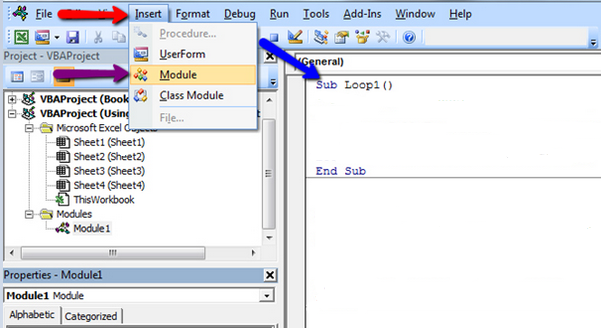Loop 1 (Displaying number with msgbox)

Sub Loop1()
Dim StartNumber As Integer
Dim EndNumber As Integer
EndNumber = 5

For StartNumber = 1 To EndNumber
MsgBox StartNumber & " is " & "Your StartNumber"
Next StartNumber

End Sub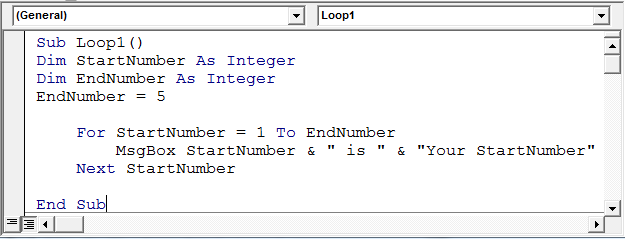Code Explanation:

• The VBA code needs you to assign value to StartNumber, where EndNumber are variables that are declared as integers as the starting point for your loop
• These values can be any number & we have EndNumber as 5
• StartNumber is started at 1
• For StartNumber = 1 To EndNumber means code will start from 1 (StartNumber) to 5 (EndNumber)
• MsgBox StartNumber & ” is ” & “Your StartNumber” will display the following message box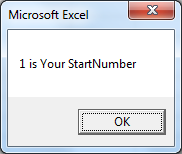Loop2 (Fill Values)

Sub Loop2()
'Fills cells A1:A56 with values of X by looping' --- Comment
'Increase value of X by 1 in each loop' --- Comment

Dim X As Integer
For X = 1 To 56
Range("A" & X).Value = X
Next X
End Sub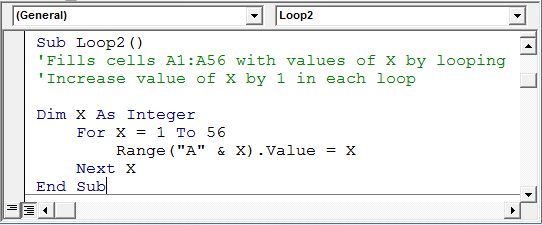Code Explanation:

• We have assigned X as an integer
• For X = 1 to 56; this will start with 1 and continue till 56 with an increment of 1 each time
• Range(“A” & X).Value = X; this line will store the value of X and will pass to range A1 to A56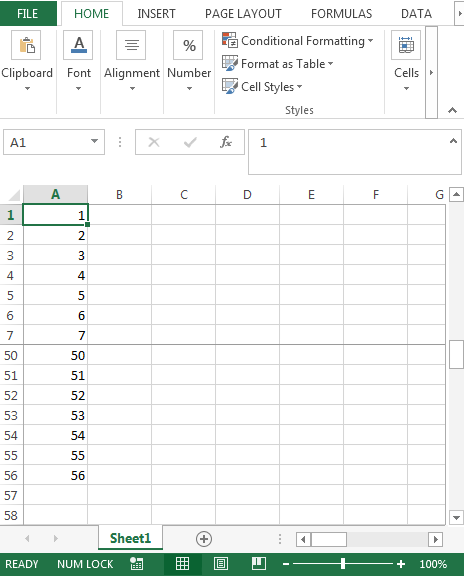Loop3 (Fill cells with background color)

Sub Loop3()
' Fills cells B1:B56 with the 56 background colours' --- Comment
Dim X As Integer
For X = 1 To 56
Range("B" & X).Select
With Selection.Interior
.ColorIndex = X
.Pattern = xlSolid
End With
Next X
End Sub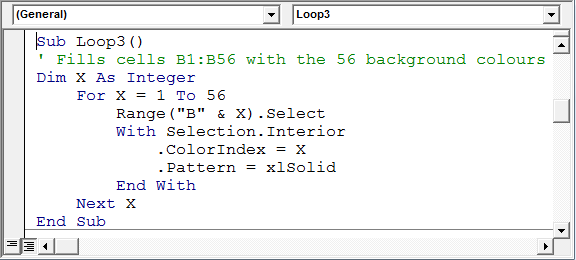Code Explanation:

• We have assigned X as an integer
• For X = 1 to 56 will start with 1 and continue till 56 with an increment of 1 each time
• Range(“B” & X).Select; this line will store the value of X & select the cell B1 till B56
• The next 4 lines i.e. With Selection.Interior will select interior colorindex & take the value from X of that colorindex such that 1 belongs to color black; 2 belongs to color white; 3 for red & so on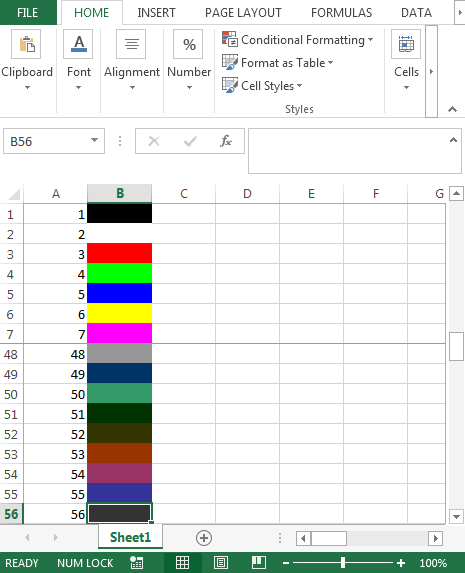Loop 4 (Fill Values with increment of 2)

By default, the Step value is forward 1, however it can be set to a number more than 1.

Sub Loop4()
' Fills every second cell from C1:C50 with values of X' --- Comment
Dim X As Integer
For X = 1 To 50 Step 2
Range("C" & X).Value = X
Next X
End Sub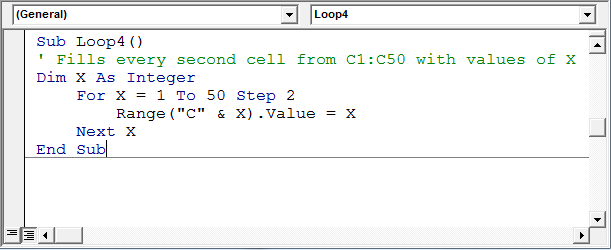Code Explanation:

• We have assigned X as an integer
• For X = 1 to 50 Step 2; this will start with 1 in X till 50 with an increment of 2 each time
• Range(“C” & X).Value = X; this line will store the value of X and will pass to range C1 to C50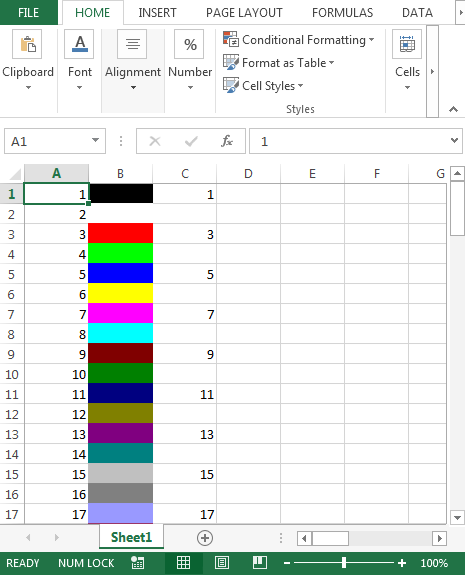Loop 5 (VBA For Loop in Reverse with STEP Instruction)

It is not necessary that counter in the For loop will only move from low to higher values; instead, For loop can run backwards, too i.e. high to lower values.

Even though the Step value is forward 1 by default, however, it can be set to a number in reverse order.

Sub Loop5()
' Fills cells from D1:D50 with values of X' --- Comment
' In this case X decreases by 1' --- Comment
Dim X As Integer, Row As Integer

Row = 1

For X = 50 To 0 Step -1
Range("D" & Row).Value = X
Row = Row + 1
Next X

End Sub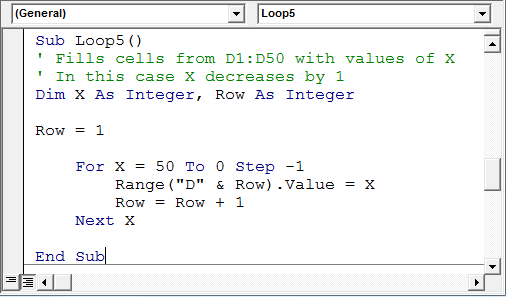Code Explanation:

• We have assigned X & Row as integer
• Row contains value 1
• For X = 50 to 0 Step -1; this will start from 50 with decrement by 1 in X till 0
• Range(“D” &Row).Value = X; this line will store the value of X and will pass to range D1 to D50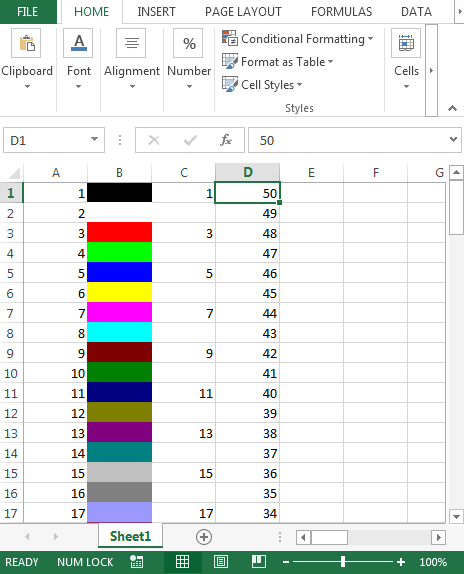Loop 6 (Fills every second cell in Reverse with STEP-2)

In the above For loop example, we can use the Step and order to see if the For loop works in forward or backward direction.

Sub Loop6()
' Fills every second cell from E1:E100 with values of X' --- Comment
' In this case X decreases by 2' --- Comment
Dim X As Integer, Row As Integer
Row = 1
For X = 100 To 0 Step -2
Range("E" & Row).Value = X
Row = Row + 2
Next X
End Sub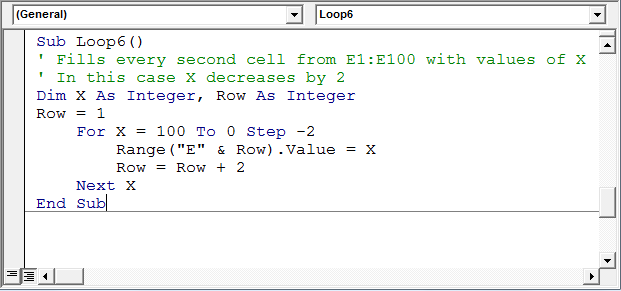Code Explanation:

• We have assigned X & Row as integer
• Row contains value 1
• For X = 100 to 0 Step -2; this will start from 100 with decrement by 2 in X till 0
• Range(“E” &Row).Value = X; this line will store the value of X and will pass to range E1 to E100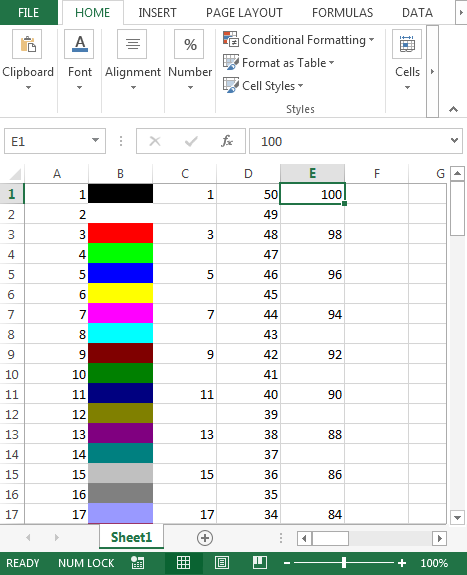Loop 7 (For Loop with IF condition: Fills cells starting from specific cell)

This will fill the cells from cell F11 with value 11 till X meets the IF condition

Sub Loop7()
' Starts to fill cells F11:F100 with values of X' --- Comment
' This will exit from the loop after 50' --- Comment

Dim X As Integer

For X = 11 To 100
Range("F" & X).Value = X
If X = 50 Then
MsgBox ("Bye Bye")
Exit For
End If
Next X

End Sub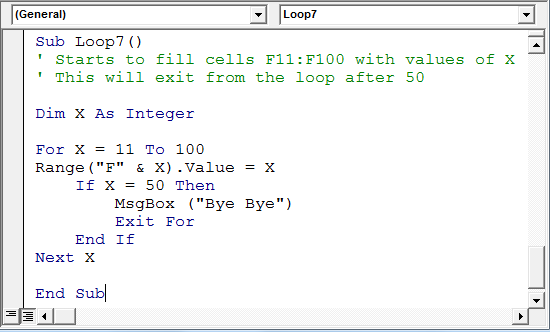Code Explanation:

• We have assigned X as an integer
• For X = 11 to 100; will start from 11 with increment by 1 in X till the condition meets
• Range(“F” &X).Value = X; this line will store the value of X and will pass to range F11 till the condition meets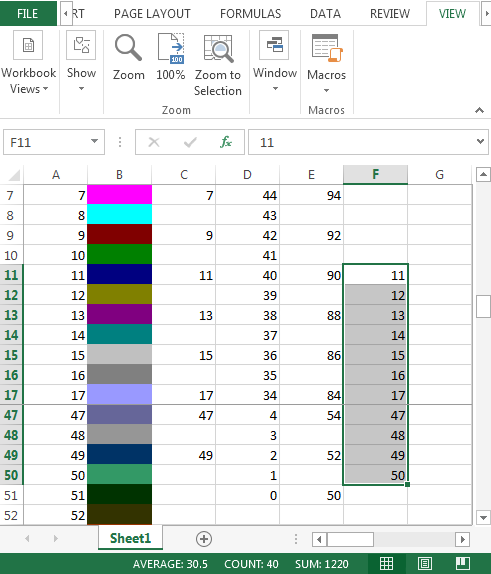• After entering the value 50 in cell F50, the following message box will be displayed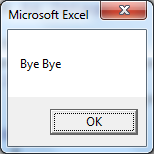Conclusion: With the above 7 examples, we can apply For loop in our regular or any automation part.

If you liked our blogs, share it with your friends on Facebook. And also you can follow us on Twitter and Facebook.
We would love to hear from you, do let us know how we can improve, complement or innovate our work and make it better for you. Write us at info@exceltip.com

Users are saying about us...

1.Hello,

Very good blog with simple & smart examples on how to use For Loops. I have bookmarked this link for future references.

Very much appreciated.

Mitchel

•Thanks Mitchel…

2.Ghanshyam singh

Nice

3.I am excited to see wonderful blog for VBA in simple terms great codes

•Thanks, V S GAUTHAMI… There’s a wave of these simple VBA explanations coming. But if you want any specific term to understand, you can mention it here.

Terms and Conditions of use

The applications/code on this site are distributed as is and without warranties or liability. In no event shall the owner of the copyrights, or the authors of the applications/code be liable for any loss of profit, any problems or any damage resulting from the use or evaluation of the applications/code.